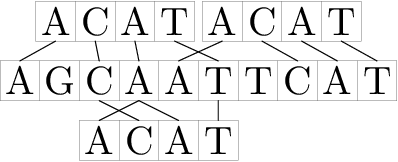CF528D Fuzzy Search

• 157通过
• 293提交
• 题目来源
• 评测方式 RemoteJudge
• 标签 快速傅里叶变换,DFT,FFT
• 难度 NOI/NOI+/CTSC
• 时空限制 3000ms / 256MB
• 提示：收藏到任务计划后，可在首页查看。

题意翻译

有两个基因串S和T，他们只包含AGCT四种字符。现在你要找出T在S中出现了几次。 有一个门限值k≥0。T在S的第i(1≤i≤|S|-|T|+1)个位置中出现的条件如下：把T的开头和S的第i个字符对齐，然后T中的每一个字符能够在S中找到一样的，且位置偏差不超过k的，那么就认为T在S的第i个位置中出现。也就是说对于所有的 j (1≤j≤|T|)，存在一个 p (1≤p≤|S|)，使得|(i+j-1)-p|≤k 和[p]=T[j]都成立。 例如，根据这样的定义"ACAT"出现在"AGCAATTCAT"的第2，3和6的位置。如果k=0，那么这个就是经典的字符串匹配问题。 现在给定门限和两个基因串S，T，求出T在S中出现的次数。

Input 单组测试数据。 第一行有三个整数 |S|,|T|,k (1≤|T|≤|S|≤200000, 0≤k≤200000)，表示S的长度，T的长度，门限值。 第二行给出基因串S。 第三行给出基因串T。 两个串都只包含大写字母'A', 'T', 'G' 和'C'。 Output 输出一个整数，表示T在S中出现的次数。

题目描述

Leonid works for a small and promising start-up that works on decoding the human genome. His duties include solving complex problems of finding certain patterns in long strings consisting of letters 'A', 'T', 'G' and 'C'.

Let's consider the following scenario. There is a fragment of a human DNA chain, recorded as a string $S$ . To analyze the fragment, you need to find all occurrences of string $T$ in a string $S$ . However, the matter is complicated by the fact that the original chain fragment could contain minor mutations, which, however, complicate the task of finding a fragment. Leonid proposed the following approach to solve this problem.

Let's write down integer $k>=0$ — the error threshold. We will say that string $T$ occurs in string $S$ on position $i$ ( $1<=i<=|S|-|T|+1$ ), if after putting string $T$ along with this position, each character of string $T$ corresponds to the some character of the same value in string $S$ at the distance of at most $k$ . More formally, for any $j$ ( $1<=j<=|T|$ ) there must exist such $p$ ( $1<=p<=|S|$ ), that $|(i+j-1)-p|<=k$ and $S[p]=T[j]$ .

For example, corresponding to the given definition, string "ACAT" occurs in string "AGCAATTCAT" in positions $2$ , $3$ and $6$ .Note that at $k=0$ the given definition transforms to a simple definition of the occurrence of a string in a string.

Help Leonid by calculating in how many positions the given string $T$ occurs in the given string $S$ with the given error threshold.

输入输出格式

输入格式：

The first line contains three integers $|S|,|T|,k$ ( $1<=|T|<=|S|<=200000$ , $0<=k<=200000$ ) — the lengths of strings $S$ and $T$ and the error threshold.

The second line contains string $S$ .

The third line contains string $T$ .

Both strings consist only of uppercase letters 'A', 'T', 'G' and 'C'.

输出格式：

Print a single number — the number of occurrences of $T$ in $S$ with the error threshold $k$ by the given definition.

输入输出样例

输入样例#1： 复制
10 4 1
AGCAATTCAT
ACAT

输出样例#1： 复制
3


说明

If you happen to know about the structure of the human genome a little more than the author of the problem, and you are not impressed with Leonid's original approach, do not take everything described above seriously.

提示
标程仅供做题后或实在无思路时参考。
请自觉、自律地使用该功能并请对自己的学习负责。
如果发现恶意抄袭标程，将按照I类违反进行处理。